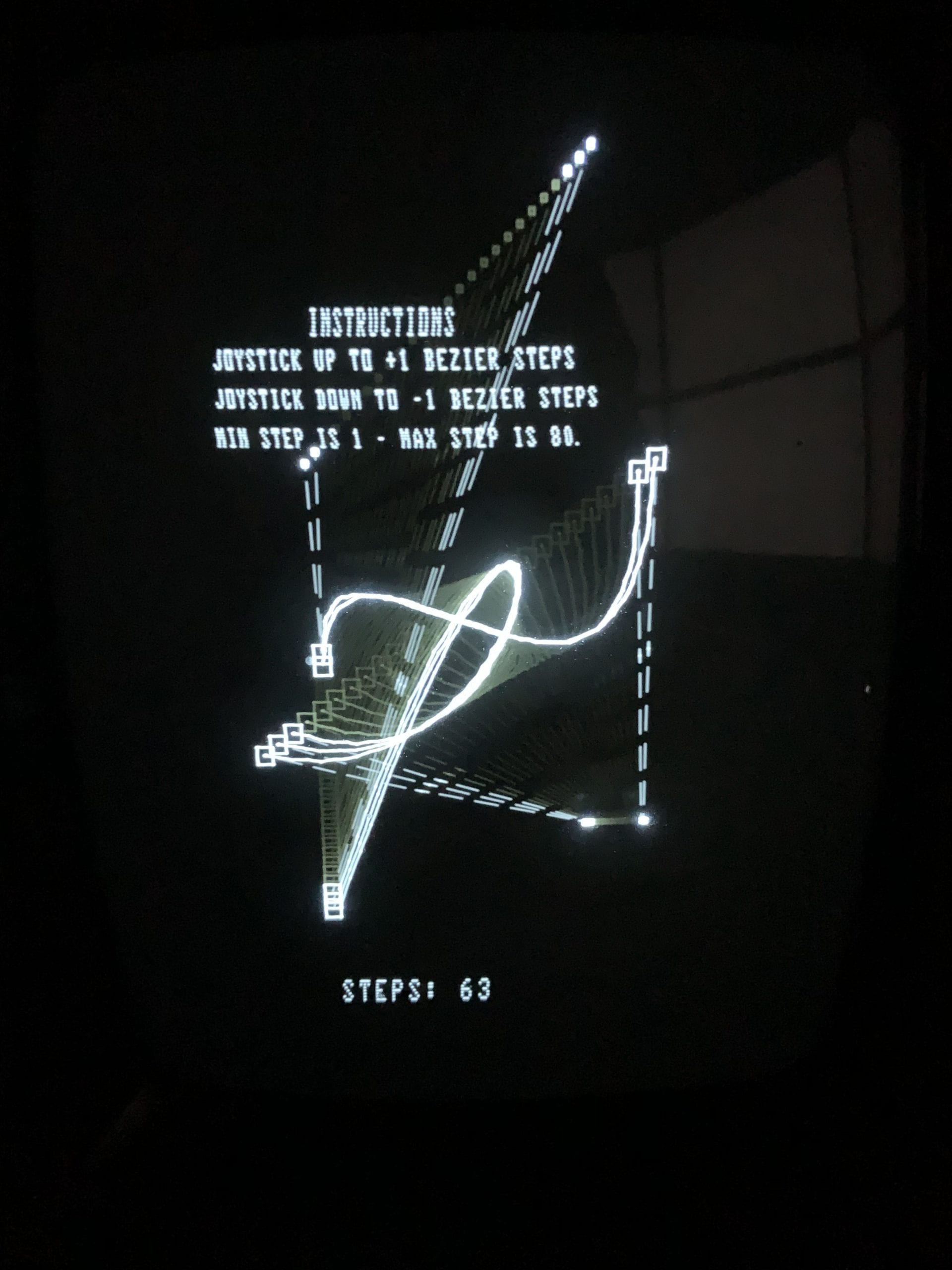While I was waiting for some equipment to continue my presentation of the Vectrex gaming console, I was looking for a simple but neat application to write today. Something that is not obvious as a game, and useful for my next post. That’s when it hit me: Bézier curves! The Vectrex BIOS has routines to display dots and segments, but nothing to handle parametric curves. And what a shame, especially if you consider that Bézier curves – a particular case of B-Splines – are at the core of modern vector packages such as vector fonts, PostScript, illustration software such as CorelDraw! or Adobe Illustrator, etc.

As you may know, Pierre Étienne Bézier is a French engineer who used these parametric curves to model car body parts at Renault. In other words, how can anyone live today without Bézier curves? So, I went ahead and used the Vectres32 cartridge to write a simple draw_bezier_curve() function that plots a cubic Bézier curve in BASIC. To illustrate the use of the function, I’ve also written a simple driver code that creates the cubic Bézier curve, moves around the anchor and control points, and accepts input from the joystick to dynamically change the number of steps used to draw the curve.

You can see a few runs of this demo in the videos and pictures attached to this post. I also added the source code so you and check it out if you are interested. I will use this code for my last post dedicated to the Vectrex console. I hope that by then, you will appreciate how advanced this little system was when it hit the US market in 1982. Enjoy!

## bezier.bas

```' bezier.bas: draws a Bezier curve, use joystip up & down top change draw step.

' Define display configuration.
scale = 80
intensity = 70
frame_rate = 20

' Define various UI configuration.
anchor_size = 4
control_size = 1
instructions = { _
{-50, 90, "INSTRUCTIONS"}, _
{-80, 80, "JOYSTICK UP TO +1 BEZIER STEPS"}, _
{-80, 70, "JOYSTICK DOWN TO -1 BEZIER STEPS"}, _
{-80, 60, "MIN STEP IS 1 - MAX STEP IS 80."} _
}

' Bn functions are respectively first, second, third and fourth
' Bernstein derivations to compute the quadratic B-Spline.
function B1(t)
return ((t) * (t) * (t))
endfunction

function B2(t)
return (3 * (t) * (t) * (1 - (t)))
endfunction

function B3(t)
return (3 * (t) * (1 - (t)) * (1 - (t)))
endfunction

function B4(t)
return ((1 - (t)) * (1 - (t)) * (1 - (t)))
endfunction

sub draw_square(x, y, s)
move = MoveSprite(x, y)
square = LinesSprite( _
{ _
{ MoveTo, s, s }, _
{ DrawTo, -s, s}, _
{ DrawTo, -s, -s }, _
{ DrawTo, s, -s }, _
{ DrawTo, s, s } _
} _
)
call ReturnToOriginSprite()
endsub

sub draw_segment(x1, y1, x2, y2)
segment = LinesSprite( _
{ _
{ MoveTo, x1, y1 }, _
{ DrawTo + \$F0, x2, y2} _
} _
)
call ReturnToOriginSprite()
endsub

sub draw_bezier_curve(x1, y1, x2, y2, x3, y3, x4, y4, s)
if s > 0.0 then
call ReturnToOriginSprite()
call draw_square(x1+anchor_size, y1, anchor_size)
call draw_square(x2, y2, control_size)
call draw_segment(x1+anchor_size, y1, x2, y2)
call draw_square(x3, y3, control_size)
call draw_square(x4, y4, anchor_size)
call draw_segment(x3, y3, x4, y4)
i = 0.0
lastx = x4
lasty = y4
d = 1.0 / s;
curve = {{ MoveTo, x1, y1 }}
repeat
x = Int(x1 * B1(i) + x2 * B2(i) + x3 * B3(i) + x4 * B4(i))
y = Int(y1 * B1(i) + y2 * B2(i) + y3 * B3(i) + y4 * B4(i))
move = {{ MoveTo, lastx, lasty}, { DrawTo, x, y }}
curve = AppendArrays(curve, move)
i = i + d
lastx = x
lasty = y
until i >= 1.0
move = {{ DrawTo, x1, y1 }}
curve = AppendArrays(curve, move)
bezier = LinesSprite(curve)
endif
endsub

' Driver code to demonstrate use of draw_bezier_curve().
x = 4
o = 80.0
s = 20
sd = 1
sil = 1
sul = 80

while true
d = 0
for i = -50.0 to 50.0 step x

' Display instructions.
textSize = {25, 4} '{40, 5}
call TextSizeSprite(textSize)
call TextListSprite(instructions)

' Display current steps.
textSize = {40, 5}
current_steps = {{-50, 150, "STEPS: " + s}}
call TextSizeSprite(textSize)
call TextListSprite(current_steps)

' Setup display for the Bezier curve.
call IntensitySprite(intensity)
call SetFrameRate(frame_rate)
call ScaleSprite(scale)

' Draw the updated Bezier curve.
call draw_bezier_curve( _
-o, -d, _
-o+d, o+d, _
o-d/4, -o, _
o-1.5*d, o-d, _
s _
)

' Prepare for next frame & update steps -- if needed.
d = d + 5
controls = WaitForFrame(JoystickDigital, Controller1, JoystickY)
if controls[1, 2] > 0 then
s = s + sd
if s > sul then
s = sul
endif
elseif controls[1, 2] < 0 then
s = s - sd
if s < sil then
s = sil
endif
endif
call ClearScreen()
call ReturnToOriginSprite()

next i
endwhile```